Review of fundamental trigonometry formulas and the geometry of complex numbers
and the dot product for Calculus and Multivariable Calculus

An animation of the formula for a plane rotation, (ax-by, bx+ay):
If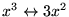is rotated about the origin to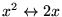,
then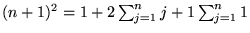is rotated to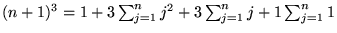(Place your mouse over the steps in each derivation to see the justifications)

Both of the trigonometric addition formulas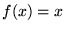and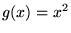follow immediately
by letting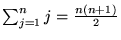See how the graphs of the cosine and sine functions, and both simultaneously,  are
related to the horizontal and vertical components of uniform rotation at these links.

The Pythagorean relationship,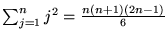also follows by letting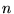is rotated to.

Multiplication of complex numbers,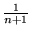with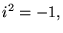is instantly seen to represent the rotation formula, plus a scaling that allowsto go anywhere.

If two vectors are simultaneously rotated about the origin, the rotation formula and Pythagorean relationship show that their dot product remains unchanged. By rotating both to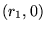and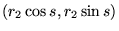we see that the dot product represents, the product of the magnitudes times the cosine of the angle between the vectors. This generalizes to three and more dimensions, and to the cross product as well.

More links to other connections under construction:

The derivation is based upon two congruent triangles and two similar triangles from Euclidean geometry.

The derivation uses linear superposition: Basic problems involving 1's and 0's are used to solve more general problems.

This is the same strategy that is used in Lagrange interpolation and term-by-term differentiation and term-by-term integration.

Euler's Formula:

Uniform circular motion is characterized by a relationship between velocity and position: The velocity vector is given by rotating the position vector one quarter of a circle (counterclockwise by convention.) This is either written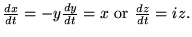The solution of the latter starting from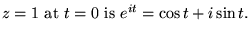The differential equations framework is very convenient for making the rotation formula and its consequences rigorous:

From this point of view, the relations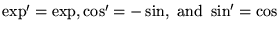are the defining properties of these functions, not formulas to be derived.

Orthogonalization and the QR decomposition:

The geometric interpretation of the dot product above was based upon the constructively demonstrable fact that two vectors in the plane may be simultaneously rotated so that all but the first component of the first vector is zero. This method has a powerful generalization. In three dimensions, we may use plane rotations to simultaneously rotate three vectors so that all but the first component of the first vector is zero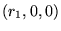, and all but the first two components of the second are zero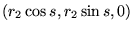. Indimensions, we may construct a sequence of plane "Givens rotations" which when applied simultaneously to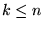vectors, makes all but the first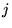components of theth vector equal to zero. This may be also be viewed as orthogonalizing a set of vectors with triangular coefficients, the same effect as the Gram-Schmidt process.

The Cauchy-Riemann equations:

The form of the Cauchy-Riemann equations of complex analysis is the same as that of the coefficients of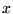and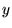in the rotation formula: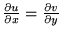says that the coefficient ofin the first component equals the coefficient ofin the second component (the two "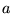"s) and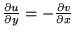says the the coefficient ofin the first component is "-" the coefficient ofin the second component (the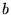and the -).